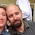I am a student teacher in my final year of University in a midsize Canadian city. I want to be a math teacher in order to break students' misconceptions about mathematics and show them how fun math can be.

## Saturday, 25 August 2012

### Modeling Data with Radical Functions

In my province we need to teach students how to take the square root of a function when given the actual function or a graph of that function (in Grade 12 Pre-Calculus Mathematics).
Why is this relevant?  When would you ever need to do this in real life?
Modeling skid marks in vehicle accidents!
This is one of the only application problems I found that incorporates taking the square root of a function for a purpose (other than to just perform the procedure or do the math).
In this document, my answers (which are hopefully correct and mathematically accurate!) are included in red/blue.
I created this worksheet/problem for students to work on as an application of radical functions because I don’t like telling students the only reason they are learning a certain topic is that it will be on the test or they will need it in later mathematics courses. Sure, every topic does not have an immediate application, but I like to use them whenever I can to keep students interested and for them to see that mathematicians discovered these concepts for a reason, not just for ‘fun’.
Here it is!  Enjoy!
PDF version

WORD version

(Hopefully the links work! If they don't, let me know :) )
Any feedback you have, whether positive or negative is greatly appreciated.  This is the first worksheet I have made about radical functions and their applications so I am not expecting it to be perfect!

#### 4 comments:

1.Taking the square root of some data, and seeing if the data then results in a nice best-fit curve is a common enough procedure, as part of attempting to match data against quadratic functions, but yeah, I can't imagine that the reverse is too terribly useful.

2.I LOVE physics applications of math content. And I completely agree -- radical functions are particularly difficult to find in the wild. I've also used the pendulum equation (http://en.wikipedia.org/wiki/Pendulum, scroll to Period of Oscillation).

Welcome to the twitterblogosphere!

3.Something that I just thought of ... what if you asked the question, if I drop an object from various heights, how long will it take to fall? This switches the independent and dependent variables from the standard set of gravity modeling calculations, and it would be a radical function!

4.Interactive online math homework help ,Best site for   math homework help solutions